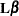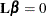PROC GLM Features

The following list summarizes the features in PROC GLM:

• PROC GLM enables you to specify any degree of interaction (crossed effects) and nested effects. It also provides for polynomial, continuous-by-class, and continuous-nesting-class effects.

• Through the concept of estimability, the GLM procedure can provide tests of hypotheses for the effects of a linear model regardless of the number of missing cells or the extent of confounding. PROC GLM displays the sum of squares (SS) associated with each hypothesis tested and, upon request, the form of the estimable functions employed in the test. PROC GLM can produce the general form of all estimable functions.

• The REPEATED statement enables you to specify effects in the model that represent repeated measurements on the same experimental unit for the same response, providing both univariate and multivariate tests of hypotheses.

• The RANDOM statement enables you to specify random effects in the model; expected mean squares are produced for each Type I, Type II, Type III, Type IV, and contrast mean square used in the analysis. Upon request, F tests that use appropriate mean squares or linear combinations of mean squares as error terms are performed.

• The ESTIMATE statement enables you to specify anvector for estimating a linear function of the parameters.

• The CONTRAST statement enables you to specify a contrast vector or matrix for testing the hypothesis that. When specified, the contrasts are also incorporated into analyses that use the MANOVA and REPEATED statements.

• The MANOVA statement enables you to specify both the hypothesis effects and the error effect to use for a multivariate analysis of variance.

• PROC GLM can create an output data set containing the input data set in addition to predicted values, residuals, and other diagnostic measures.

• PROC GLM can be used interactively. After you specify and fit a model, you can execute a variety of statements without recomputing the model parameters or sums of squares.

• For analysis involving multiple dependent variables but not the MANOVA or REPEATED statements, a missing value in one dependent variable does not eliminate the observation from the analysis for other dependent variables. PROC GLM automatically groups together those variables that have the same pattern of missing values within the data set or within a BY group. This ensures that the analysis for each dependent variable brings into use all possible observations.

• The GLM procedure automatically produces graphs as part of its ODS output. For general information about ODS Graphics, see the section ODS Graphics and Chapter 21: Statistical Graphics Using ODS.